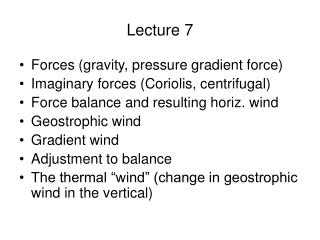Download PresentationLecture 7Lecture 7 - PowerPoint PPT Presentation

Download PresentationLecture 7
An Image/Link below is provided (as is) to download presentation

Download Policy: Content on the Website is provided to you AS IS for your information and personal use and may not be sold / licensed / shared on other websites without getting consent from its author. While downloading, if for some reason you are not able to download a presentation, the publisher may have deleted the file from their server.

- - - - - - - - - - - - - - - - - - - - - - - - - - - E N D - - - - - - - - - - - - - - - - - - - - - - - - - - -
Presentation Transcript

1. Lecture 7 • Forces (gravity, pressure gradient force) • Imaginary forces (Coriolis, centrifugal) • Force balance and resulting horiz. wind • Geostrophic wind • Gradient wind • Adjustment to balance • The thermal “wind” (change in geostrophic wind in the vertical)

2. Wind direction is the direction from which the wind is blowing. Wind direction is expressed in degrees Units: m/s or knots 1 knot = 0.5 m/s 1knot ~ 1 mile/hr Wind speed is expressed in terms of the flagpole

3. Newton’s law of motion • A body at rest tends to stay at rest; a body in motion tends to stay in motion, traveling at a constant speed in a straight line. • A force exerted on a body of mass m causes the body to accelerate in the direction of the applied force. Force = mass x acceleration

4. A force has direction and magnitude (it is a vector). Adding two forces is vector addition.

5. Forces that move the air • Gravitational force (g=9.8 m/s2) • Pressure gradient force (-1/rho x dp/dx or --1/rho x dp/dy). It points toward lower p. The pressure gradients causing the wind are horizontal. • Coriolis force • Centrifugal force • Frictional force

6. Pressure gradient force pushes from higher to lower pressures PGF Magnitude depends on value of pressure gradient

7. Pressure (p) as a function of height

8. Vertical structure in the atmosphere • What about pressure? • Hydrostatic equation: balance between pressure gradient force and gravity. • dp/dz = - rho g • Ideal gas law: • p = rho R T Let’s go to the board! z = - H ln (p/p0), where H is scale height and is only constant if T is constant. In other words, p = p0 exp(- z/H)

9. Next: Coriolis forceEarth’s rotational speed is greatest at the equator and exactly zero at the poles

10. Coriolis deflection Coriolis force deflects moving air to the right in the Northern Hemisp. Coriolis force deflects moving air to the left in the S. Hemisphere

11. The magnitude of the Coriolis force (CF) is proportional to the wind speed and sine of latitude CF= f x V, Where f is 2xEarth’s rotation rate xsin(latitude)

12. Centrifugal force: arises because the trajectory is curved. CENTF= V^2/R, where R (radius of curvature) is positive for cyclones, negative for anticyclones

13. Frictional force is proportional to the wind- speed and directed opposite to the wind dir. FF = -k V (where k describes the roughness)

14. Summary: The Forces • Gravity – the strong silent type • PGF arises from pressure gradients generated by differential solar heating –leads to wind. Only then do the other forces start acting. • CF, CENTF, FF all depend of V, wind speed.

15. Atmospheric force balancesSum of forces = mass x accelerationBalance when the forces add up to zero • Hydrostatic balance in the vertical (gravity does not cause wind). • Strong horizontal PGF means strong wind • CF changes wind direction not speed • CENTF only acts on curved flow • FF slows down the wind (regardless of direction).

16. Geostrophic wind, geostrophic balancePGF + CF = 0

17. Wind blows counterclockwise around lows (cyclonic wind in cyclones), clockwise around highs (anticyclones) Force balance not quite right since we have curved flows Add centrifugal force

18. Gradient balance and the gradient wind

19. Gradient balance results in gradient wind • Represents balance of three forces • It is an excellent approximation to free atmospheric flow. • Around highs it is supergeostrophic • Around lows it is subgeostrophic

20. Adjustment to balance • The atmosphere tries hard to stay in balance, but it is constantly being pushed away from it. • The atmosphere adjusts very quickly (in a matter of minutes) to imbalance.

21. Adjustment to balance with 3 forces: